TitleCollege Algebra
Tutorial 35: Graphs of Polynomial
FunctionsLearning Objectives

 After completing this tutorial, you should be able to: Identify a polynomial function. Use the Leading Coefficient Test to find the end behavior of the graph of a given polynomial function. Find the zeros of a polynomial function. Find the multiplicity of a zero and know if the graph crosses the x-axis at the zero or touches the x-axis and turns around at the zero. Know the maximum number of turning points a graph of a polynomial function could have. Graph a polynomial function.Introduction

 In this tutorial we will be looking at graphs of polynomial functions.  If you need a review on functions, feel free to go to Tutorial 30: Introduction to Functions.  If you need a review on polynomials in general, feel free to go to Tutorial 6: Polynomials.  Basically, the graph of a polynomial function is a smooth continuous curve.  There are several main aspects of this type of graph that you can use to help put the curve together.  I will be going over how to use the leading term of your polynomial function to determine the end behavior of its graph.  We will also be looking at finding the zeros, aka the x-intercepts, as well as the y-intercept of the graph. If you need a review on intercepts, feel free to go to Tutorial 26: Equations of Lines.  Another important concept is to know the largest possible number of  turning points.  This will help you be more accurate in the graph that you draw.  That just about covers it.  I guess you are ready to get to it.Tutorial

 Polynomial Function A polynomial function is a function that can be written in the form, whereare real numbers and n is a nonnegative integer.

 Basically it is a function whose rule is given by a polynomial in one variable. If you need a review on functions, feel free to go to Tutorial 30: Introduction to Functions.  If you need a review on polynomials in general, feel free to go to Tutorial 6: Polynomials. An example of a polynomial function is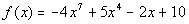.

 Leading Term  When the polynomial function is written in standard form,, the leading term is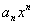.

 In other words, the leading term is the term that the variable has its highest exponent. The leading term of the functionwould be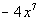.

 Leading Coefficient  When the polynomial function is written in standard form,, the leading coefficient is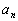.

 Basically, the leading coefficient is the coefficient on the leading term. The leading coefficient of the functionwould be - 4.

 Degree of a Term of a Polynomial Function

 The degree of a term of a polynomial function is the exponent on the variable.

 Degree of a Polynomial Function When the polynomial function is written in standard form,, the degree of the polynomial function is n.

 The degree of the polynomial is the largest degree of all of its terms. The degree of the functionwould be 7.

 There are four cases that go with this test:
Given a polynomial function in standard form:

 Case 1: If n is odd AND the leading coefficient, is positive, the graph falls to the left and rises to the right: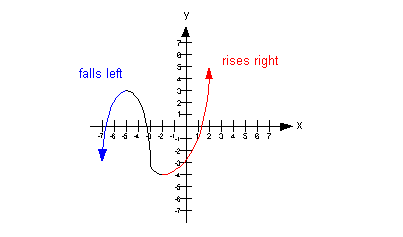Case 2: If n is odd AND the leading coefficient, is negative, the graph rises to the left and falls to the right.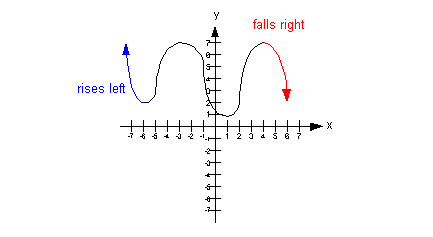Case 3: If n is even AND the leading coefficient, is positive, the graph rises to the left and to the right.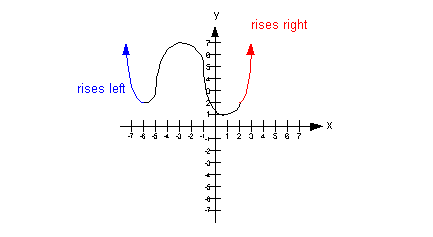Case 4: If n is even AND the leading coefficient, is negative, the graph falls to the left and to the right.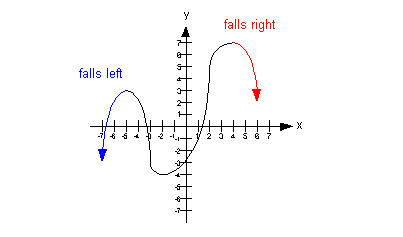Example 1: Use the Leading Coefficient Test to determine the end behavior of the graph of the polynomial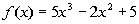.

 First question is what is the leading term? If you said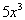, you are correct!!   Second question is what is the leading term’s degree? If you said 3, you are right on!!  3 is the exponent on the leading term, which also means it is the degree of the polynomial.   Third question is what is the coefficient on the leading term? If you said 5, pat yourself on the back!!   Putting this information together with the Leading Coefficient Test we can determine the end behavior of the graph of our given polynomial: Since the degree of the polynomial, 3, is odd and the leading coefficient, 5, is positive, then the graph of the given polynomial falls to the left and rises to the right.Example 2: Use the Leading Coefficient Test to determine the end behavior of the graph of the polynomial.

 First question is what is the leading term? If you said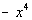, you are correct!!   Second question is what is the leading term’s degree? If you said 4, you are right on!!  4 is the exponent on the leading term, which also means it is the degree of the polynomial.   Third question is what is the coefficient on the leading term? If you said -1, pat yourself on the back!!   Putting this information together with the Leading Coefficient Test we can determine the end behavior of the graph of our given polynomial: Since the degree of the polynomial, 4,  is even and the leading coefficient, -1, is negative, then the graph of the given polynomial falls to the left and falls to the right.Example 3: Use the Leading Coefficient Test to determine the end behavior of the graph of the polynomial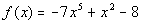.

 First question is what is the leading term? If you said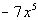, you are correct!!   Second question is what is the leading term’s degree? If you said 5, you are right on!!  5 is the exponent on the leading term, which also means it is the degree of the polynomial.   Third question is what is the coefficient on the leading term? If you said -7, pat yourself on the back!!   Putting this information together with the Leading Coefficient Test we can determine the end behavior of the graph of our given polynomial: Since the degree of the polynomial, 5,  is odd and the leading coefficient, -7, is negative, then the graph of the given polynomial rises to the left and falls to the right.Example 4: Use the Leading Coefficient Test to determine the end behavior of the graph of the polynomial.

 First question is what is the leading term? If you said, you are correct!!   Second question is what is the leading term’s degree? If you said 6, you are right on!!  6 is the exponent on the leading term, which also means it is the degree of the polynomial.   Third question is what is the coefficient on the leading term? If you said 1, pat yourself on the back!!   Putting this information together with the Leading Coefficient Test we can determine the end behavior of the graph of our given polynomial: Since the degree of the polynomial, 6,  is even and the leading coefficient, 1, is positive, then the graph of the given polynomial rises to the left and rises to the right.

 Zeros (or Roots) of Polynomial Functions

 A zero or root of a polynomial function is the value of x such that f(x) = 0.  In other words it is the x-intercept, where the functional value or y is equal to 0.

 Zero of Multiplicity k If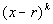is a factor of a polynomial function f and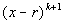is not a factor of f, then r is called a zero of  multiplicity k of f.

 In other words, when a polynomial function is set equal to zero and has been completely factored and each different factor is written with the highest appropriate exponent, depending on the number of times that factor occurs in the product, the exponent on the factor that the zero is a solution for, gives the multiplicity of that zero.  The exponent indicates how many times that factor would be written out in the product, this gives us a multiplicity.

 Multiplicity of Zeros and the x-intercept

There are two cases that go with this concept:

 Case 1: If r is a zero of even multiplicity: This means the graph touches the x-axis at r and turns around. This happens because the sign of f(x) does not change from one side to the other side of r.

 Case 2: If r is a zero of odd multiplicity: This means the graph crosses the x-axis at r. This happens because the sign of f(x) changes from one side to the other side of r.

 Turning Points If f is a polynomial function of degree n, then  there is at most n - 1 turning points on the graph of f.

 A turning point is a point at which the graph changes direction. Keep in mind that you can have fewer than n - 1 turning points, but it will never exceed n - 1 turning points.Example 5: Find the zeros for the polynomial function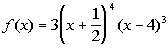and give the multiplicity for each zero.  Indicate whether the graph crosses the x-axis or touches the x-axis and turns around at each zero.

 First Factor: The first factor is 3, which is a constant.  Therefore, there are no zeros that go with this factor.

 Second Factor:*Setting the 2nd factor = 0 *Solve for x *x = -1/2 is a zero

 What would the multiplicity of the zero x = -1/2 be? If you said the multiplicity for x = -1/2 is 4, you are correct!!!!  Since the exponent on this factor is 4, then its multiplicity is 4.   Does the graph cross the x-axis or touch the x-axis and turn around at the zero x = -1/2? If you said it touches the x-axis and turns around at the zero x = -1/2, pat yourself on the back!!!  It does this because the multiplicity is 4, which is even.

 Third Factor: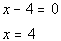*Setting the 3rd factor = 0 *Solve for x *x = 4 is a zero

 What would the multiplicity of the zero x = 4 be? If you said the multiplicity for x = 4 is 3, you are correct!!!!  Since the exponent on this factor is 3, then its multiplicity is 3.   Does the graph cross the x-axis or touch the x-axis and turn around at the zero x = 4? If you said it crosses the x-axis at the zero x = 4, pat yourself on the back!!!  It does this because the multiplicity is 3, which is odd.Example 6: Find the zeros for the polynomial function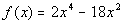and give the multiplicity for each zero.  Indicate whether the graph crosses the x-axis or touches the x-axis and turns around at each zero.

 Let’s factor this one first: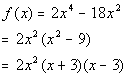*Factor out a GCF *Factor a diff. of squares

 First Factor: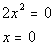*Setting the 1st factor = 0 *Solve for x *x = 0 is a zero

 What would the multiplicity of the zero x = 0 be? If you said the multiplicity for x = 0 is 2, you are correct!!!!  Since the exponent on this factor is 2, then its multiplicity is 2.   Does the graph cross the x-axis or touch the x-axis and turn around at the zero x = 0? If you said it touches the x-axis and turns around at the zero x = 0, pat yourself on the back!!!  It does this because the multiplicity is 2, which is even.

 Second Factor: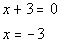*Setting the 2nd factor = 0 *Solve for x *x = -3 is a zero

 What would the multiplicity of the zero x = -3 be? If you said the multiplicity for x = -3 is 1, you are correct!!!!  Since the exponent on this factor is 1, then its multiplicity is 1.   Does the graph cross the x-axis or touch the x-axis and turn around at the zero x = -3? If you said it crosses the x-axis at the zero x = -3, pat yourself on the back!!!  It does this because the multiplicity is 1, which is odd.

 Third Factor: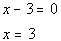*Setting the 3rd factor = 0 *Solve for x *x = 3 is a zero

 What would the multiplicity of the zero x = 3 be? If you said the multiplicity for x = 3 is 1, you are correct!!!!  Since the exponent on this factor is 1, then its multiplicity is 1.   Does the graph cross the x-axis or touch the x-axis and turn around at the zero x = 3? If you said it crosses the x-axis at the zero x = 3, pat yourself on the back!!!  It does this because the multiplicity is 1, which is odd.

 Graphing a Polynomial Function

 Step 1: Determine the graph’s end behavior.

 Use the Leading Coefficient Test, described above, to find if the graph rises or falls to the left and to the right.

 Step 2: Find the x-intercepts or zeros of the function.

Recall that you find your x-intercept or zero by setting your function equal to 0, f(x) = 0, completely factoring the polynomial and setting each factor equal to 0.

If you need a review on x-intercepts, feel free to go to Tutorial 26: Equations of Lines.

Keep in mind that whenis a factor of your polynomial and

 a) if k is even, the graph touches the x-axis at r and turns around. b) if k is odd, the graph crosses the x-axis at r.

 Step 3: Find the y-intercept of the function.

 Recall that you can find your y-intercept by letting x = 0 and find your functional value at x = 0, f(0). If you need a review on y-intercepts, feel free to go to Tutorial 26: Equations of Lines.

 Step 4: Determine if there is any symmetry.

 y-axis symmetry: Recall that your function is symmetric about the y-axis if it is an even function.  In other words, if f(-x) = f(x), then your function is symmetric about the y-axis. Origin symmetry: Recall that your function is symmetric about the origin if it is an odd function.  In other words, if  f(-x) = -f(x), then your function is symmetric about the origin. If you need a review on even and odd functions, feel free to go to Tutorial 32: Graphs of Functions, Part II.

 Step 5: Find the number of maximum turning points.

 As discussed above, if f is a polynomial function of degree n, then there is at most n - 1 turning points on the graph of f.

 Step 6: Find extra points, if needed.

 Sometimes you may need to find points that are in between the ones you found in steps 2 and 3 to help you be more accurate on your graph.

 Step 7: Draw the graph.

 Plot the points found in steps 2, 3, and 6 and use the information gathered in steps 1, 2, 4, and 5 to draw your graph. The graph of polynomial functions is always a smooth continuous curve.Example 7: Given the polynomial function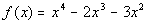a) use the Leading Coefficient Test to determine the graph’s end behavior, b) find the x-intercepts (or zeros) and state whether the graph crosses the x-axis or touches the x-axis and turns around at each x-intercept, c) find the y-intercept, d) determine the symmetry of the graph, e) indicate the maximum possible turning points, and f) graph.

 Step 1: Determine the graph’s end behavior.

 Use the Leading Coefficient Test, described above, to find if the graph rises or falls to the left and to the right. Do you think that the graph rises or falls to the left and to the right? Since the degree of the polynomial, 4,  is even and the leading coefficient, 1, is positive, then the graph of the given polynomial rises to the left and rises to the right.

 Step 2: Find the x-intercepts or zeros of the function.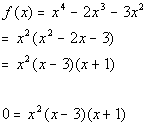*Factor out a GCF *Factor a trinomial

 First Factor: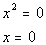*Setting the 1st factor = 0 *Solve for x *x = 0 is a zero

 Since the exponent on this factor is 2, then the multiplicity for the zero x = 0 is 2.   Since the multiplicity is 2, which is even, then the graph touches the x-axis and turns around at the zero x = 0.

 Second Factor:*Setting the 2nd factor = 0 *Solve for x *x = 3 is a zero

 Since the exponent on this factor is 1, then the multiplicity for the zero x = 3 is 1.   Since the  multiplicity is 1, which is odd, then the graph crosses the x-axis at the zero x = 3.

 Third Factor:*Setting the 3rd factor = 0 *Solve for x *x = -1 is a zero

 Since the exponent on this factor is 1, then the multiplicity for the zero x = -1 is 1. Since the multiplicity is 1, which is odd, then the graph crosses the x-axis at the zero x = -1.

 Step 3: Find the y-intercept of the function.

 Letting x = 0 we get:*Plug in 0 for x

 The y-intercept is (0, 0).

 Step 4: Determine if there is any symmetry.

 y-axis symmetry: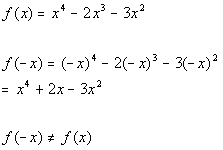*Plug in -x for x

 It is not symmetric about the y-axis.

 Origin symmetry:*Plug in -x for x   *Take the opposite of f(x)

 It is not symmetric about the origin.

 Step 5: Find the number of maximum turning points.

 Since the degree of the function is 4, then there is at most 4 - 1 = 3 turning points.

 Step 6: Find extra points, if needed.

 To get a more accurate curve, lets find some points that are in between the points we found in steps 2 and 3:

 x(x, y) -.5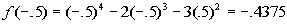(-.5, -.437) 1(1, -4) 2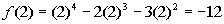(2, -12)

 Step 7: Draw the graph.Example 8: Given the polynomial function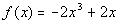a) use the Leading Coefficient Test to determine the graph’s end behavior, b) find the x-intercepts (or zeros) and state whether the graph crosses the x-axis or touches the x-axis and turns around at each x-intercept, c) find the y-intercept, d) determine the symmetry of the graph, e) indicate the maximum possible turning points, and f) graph.

 Step 1: Determine the graph’s end behavior.

 Use the Leading Coefficient Test, described above, to find if the graph rises or falls to the left and to the right. Do you think that the graph rises or falls to the left and to the right? Since the degree of the polynomial, 3,  is odd and the leading coefficient, -2, is negative, then the graph of the given polynomial rises to the left and falls to the right.

 Step 2: Find the x-intercepts or zeros of the function.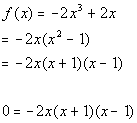*Factor out a GCF *Factor a diff. of squares

 First Factor: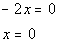*Setting the 1st factor = 0 *Solve for x *x = 0 is a zero

 Since the exponent on this factor is 1, then the multiplicity for the zero x = 0 is 1. Since the  multiplicity is 1, which is odd, then the graph crosses the x-axis at the zero x = 0.

 Second Factor: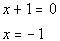*Setting the 2nd factor = 0 *Solve for x *x = -1 is a zero

 Since the exponent on this factor is 1, then the multiplicity for the zero x = -1 is 1.   Since the  multiplicity is 1, which is odd, then the graph crosses the x-axis at the zero x = -1.

 Third Factor: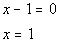*Setting the 3rd factor = 0 *Solve for x *x = 1 is a zero

 Since the exponent on this factor is 1, then the multiplicity for the zero x = 1 is 1. Since the multiplicity is 1, which is odd, then the graph crosses the x-axis at the zero x = 1.

 Step 3: Find the y-intercept of the function.

 Letting x = 0 we get: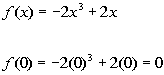*Plug in 0 for x

 The y-intercept is (0, 0).

 Step 4: Determine if there is any symmetry.

 y-axis symmetry:*Plug in -x for x

 It is not symmetric about the y-axis.

 Origin symmetry: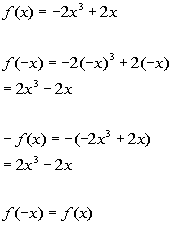*Plug in -x for x   *Take the opposite of f(x)

 It is symmetric about the origin.

 Step 5: Find the number of maximum turning points.

 Since the degree of the function is 3, then there is at most 3 - 1 = 2 turning points.

 Step 6: Find extra points, if needed.

 To get a more accurate curve, lets find some points that are in between the points we found in steps 2 and 3:

 x(x, y) -1/2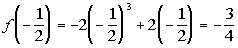(-1/2, -3/4) 1/2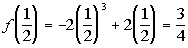(1/2, 3/4)

 Step 7: Draw the graph.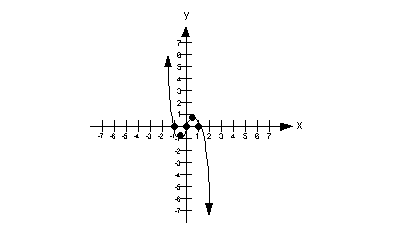Practice Problems

 These are practice problems to help bring you to the next level.  It will allow you to check and see if you have an understanding of these types of problems. Math works just like anything else, if you want to get good at it, then you need to practice it.  Even the best athletes and musicians had help along the way and lots of practice, practice, practice, to get good at their sport or instrument.  In fact there is no such thing as too much practice. To get the most out of these, you should work the problem out on your own and then check your answer by clicking on the link for the answer/discussion for that  problem.  At the link you will find the answer as well as any steps that went into finding that answer.Practice Problems 1a - 1b: Given the polynomial function a) use the Leading Coefficient Test to determine the graph’s end behavior, b) find the x-intercepts (or zeros) and state whether the graph crosses the x-axis or touches the x-axis and turns around at each x-intercept, c) find the y-intercept, d) determine the symmetry of the graph, e) indicate the maximum possible turning points, and f) graph.

 1a.(answer/discussion to 1a) 1b.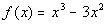(answer/discussion to 1b)Need Extra Help on these Topics?

 Go to Get Help Outside the Classroom found in Tutorial 1: How to Succeed in a Math Class for some more suggestions.

Last revised on March 14, 2012 by Kim Seward.